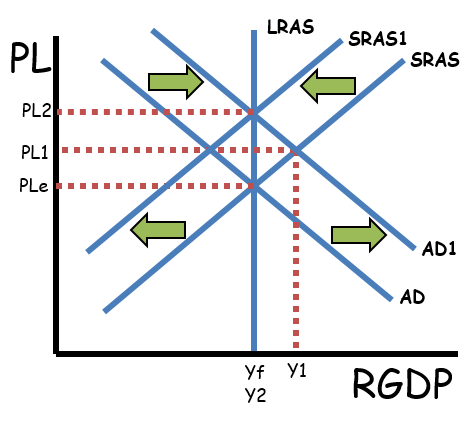### ReviewEcon.com

AP, IB, and College Microeconomic and Macroeconomic Principles

# The Quantity Theory of Money

11/18/2021 Jacob Reed
Famous Economist Milton Friedman said, “Inflation is always and everywhere a monetary phenomenon.” The quantity theory of money and the monetary equation of exchange help us understand what Mr. Friedman was getting at. This monetarist economic theory helps us understand how changes in the money supply can impact the short-run and long-run macro-economy.1. What are the long-run consequences of an increase in the money supply?

As you learned in the section about monetary policy, increases in the money supply cause a decrease in the interest rate, increase gross investment, and shift the aggregate demand curve to the right in the short run. That rightward shift of the aggregate demand curve, indicates that an increase in the money supply will cause an increase in real output.

In the long-run however, the rightward shift of the aggregate demand curve will cause an increase in wages and resource prices. That will cause a leftward shift of the short-run aggregate supply curve, returning the economy to the previous level of real output with higher price levels. That means the long-run impact of an increase in the money supply is an increase in the price level with no change in real output.

Note: This long-run impact of an increase in the money supply is best understood when starting from long-run equilibrium.2. What is the monetary equation of exchange (quantity theory of money formula)?

The Equation: M x V = P x Y = Nominal GDP
The variables in the monetary equation of exchange are:
M = Money Supply
V = Velocity of money (how many times a dollar is spent in a year)
P = Price level
Y = Real output (rGDP)
(Both M x V and P x Y equal nominal GDP.)

3. What are the implications of the monetary equation of exchange?

If the velocity of money is stable (V), the money supply must increase when there are increases in real GDP (Y) if prices are to remain stable (P).  Also, increases in the money supply (while the velocity is stable) without concurrent increases in output will cause inflation (P rises). While the velocity of money is always not stable (see the Federal Reserve’s M1 Velocity), the equation still holds true.

The quantity theory of money tells us that if the money supply increases too quickly, inflation will result. If the money supply decreases, deflation will result. Also, if the economy is already at full employment, increases in the money supply will not change real output in the long run; they will only cause inflation.